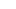# CAT Tricky Questions – How to Solve and find Answers in a minuteAre tricky CAT questions getting to you? Stuck with them all day? Even these can be dealt with in a snap! Stated below are some examples of CAT problems that you may need to look into. Remember these when you face these trick questions in your CAT examination and things are likely to become way easier for you!

• Probability

It is one of the trickiest (as well as fascinating) topics in the CAT quantitative aptitude section. This topic can present tricky problems that will test a combination of your mathematical and reasoning skills at the same time. A couple of examples are provided below to give you a better picture:

Example 1: One ticket is randomly selected from a bunch of 50 tickets numbered  0, 01, 02, ……, 49.   In that case, 8  is the probability of  the sum of the digits on the selected ticket, given that the product of these digits is zero equals

(a) 1/14
(b) 1/7
(c) 5/14
(d) 1/50

Solution: (a)

Let A be the event that the sum of the digits on the selected ticket is 8, then

B is the event that the product of the digits is zero.

Example 2: It is given that the events A and B are such that P(A) = ¼, P(A|B) = ½ and P(B|A) = 2/3. Then P(B|A) = 2/3. Then P(B) is
(a) 1/2

(b) 1/6
(c) 1/3
(d) 2/3

Solution: (c)

Next comes the quantitative section of CAT. An example of two different types of quantitative problems each has been provided keeping a note of the challenges that the candidate might have to face.

Example 1: Sam bought ‘a’ books for ‘b’ rupees, where ‘a’ and ‘b’ are integers. If he had bought 10 more books he would have got all of them for Rs. 2 and saved 80 paisa per dozen. What are the respective vales of ‘a’ and ‘b’?

1. 3,2
2. 6,1
3. 5,1
4. 4,2

Approach: a books are for b rupees; and a+10 books are for 2 rupees
Therefore, b < 2 and since b is an integer, so b=1
(100/a) – (200/a+10) = 80/12
a = 5

### Last Minute Tips for CAT 2019

Challenge: Ability to determine the value of ‘b’ and moving on

Example 2: The integers 49966 and 52231 when divided by a three digit number ‘n’ produce the same remainder. Find out the value of n.

1. 453
2. 298
3. 462
4. 367

Topic: Numbers (Remainders)

Approach: 52231-49966=2265

2265 should be divisible by the three-digit number (if x=kn+m, y=ln+m, the remainder

will be m when x and y are divided by n, then x-y= n(k-l)

Now, 2265 = 453*5

Thus, n=453

Challenge: Understanding that the difference between two natural numbers can be

divided by the same divisor also.

Stay connected with fellow students on PaGaLGuY for CAT 2019 Preparation

• General Knowledge can also challenge you with brain teasers and riddles. Here are a few examples to help you with it:

How many Dynes are there in 1 gram weight?

1. 900
B. 250
C. 375
D. 981

Solution: Option D, i.e., 98

The dyne is a derived unit of force that is specified in the centimeter–gram–second (CGS) system of units which is a predecessor of the modern SI unit.

Example 2: If 5 people can build 5 houses in only 5 days, how long will it take 100 people to build 100 houses?

Solution: It will take 100 people only 5 days for building 100 houses.

1 person can build a house 1 house in 5 days.

Thus, when 100 people are working simultaneously, they will build 100 houses in 5 days.

See? It is as simple. All you need is a steady mind to analyze the question, get the catch and twist around to find the solution accordingly. Hope you ace the exam!

Remember to eat healthy and stay fit!

Best of luck!